## General Mechanical

Topics relate to Mechanical Enterprise, Motion, Additive Print and more

•Shivani
Subscriber

Hi!

I am trying to simulate a model which is a mining structure.I have to simulate it for forces that already exist in the structure(in-situ stresses).The pressure varies at each point with respect to change in height (vertically and horizontally),uniformly.I am using magnitude (function) to define my boundary condition for the pressure.I am trying to simulate it in workbench but haven't reached the expected result.Will I have to incorporate APDL commands for my uniformly varying load?Is this kind of simulation possible in workbench?

I am an absolute newcomer to ansys and am stuck with what to do next with the solution.

•peteroznewman
Subscriber

Hi,

Here is an example from the Cornell edX course that has an example of a pressure that varies as a function of the spatial z coordinate.

https://youtu.be/0e1YsV9pmUA

•Shivani
Subscriber

Thank you.

That was helpful.But I'm looking for application of pressure in all three directions (x,y,z) simultaneously,just the way it is done for direction z.Because when I do that, my results don't add up.

Thank you for the video,it is very helpful.

•peteroznewman
Subscriber

How does the pressure vary in x,y,z ?
Is it an equation that is a function of the spatial coordinates?
I also want the geometry of the surface to which the pressure is being applied.

One way to simplify a complicated BC is to move the boundary out and model the structure that causes the complicated BC on the smaller model in a larger model. Then it may be possible to apply a much simpler BC to the larger model and the solution will create the pressure on the surface for you.

•Shivani
Subscriber

Following are the equations governing the change in pressure in respective directions:

z direction: 25000*(200+z)MPa

x&y direction: (2.4+.01*y)*1000000 MPa

These are the equations for a structure 200mtr below the surface of earth.And the pressure varies at each point.I'm attaching the picture of the structure.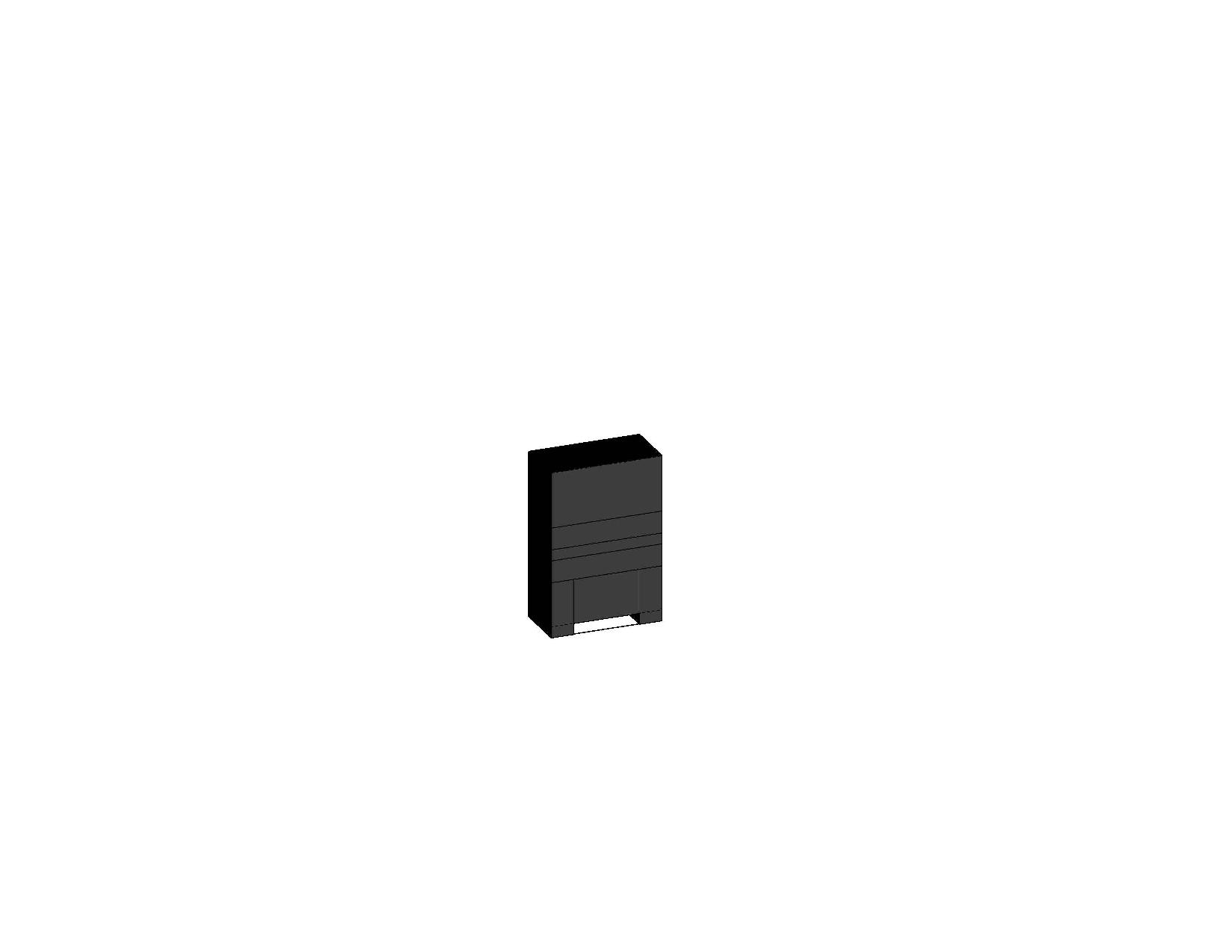The dimensions of the geometry being :

height=19mtr

width=5mtr

length=16mtr.

Face(both sides) 16*5 and  5*19 experiencing the pressure according to equation of x and y direction.

The top face experiencing the pressure according to equation of z direction with the bottom most layer being fixed.

And I did not understand "model the structure that causes the complicated BC on the smaller model in a larger model. Then it may be possible to apply a much simpler BC to the larger model and the solution will create the pressure on the surface for you ".

Thank you for your time and help,it is really a very big help.

•peteroznewman
Subscriber

"model the structure that causes the complicated BC on the smaller model in a larger model. Then it may be possible to apply a much simpler BC to the larger model and the solution will create the pressure on the surface for you ".

Something causes the change in pressure. Instead of using a formula to create the variation in pressure, add the thing that is causing the pressure to the model. That thing may be simpler to apply BCs to, than the formula for the pressure.

I will come back later to see if I can find a way to use that equation, but it would be helpful if you upload an archive of your model. You can attach .wbpz files to your posts using the Attach button as long as they are < 120 MB.

•sikandar_iit_ism
Subscriber

Hey Shivani,

well, I am also working the same type of work in an underground excavation but also I am a newcomer to ansys workbench.

•Shivani
Subscriber

Hi @sikandat_iit_ism

We could actually meet and discuss the problem.I am having alot of troubles.

•Shivani
Subscriber

The pressure as I had mentioned earlier is the in-situ stress,ie they are natural geographical forces always present in the rock.

And I shall attach the model in the next post,thank you.

•Shivani
Subscriber

I have attached file.

Please refer to it.And I also want to add to the information that the pressure that I'm applying,I want to do it for the whole body but I'm only able to apply it on a surface so wanted to know if the pressure is transmitted across the whole structure or not.And what would be the difference between surface and direct effect in application of pressure.

And in the final analysis I want the maximum stress to be at the bottom of the structure(because pressure is a function of distance here
) which I am not able to get.Please let me know what all corrections do I need to make.

•peteroznewman
Subscriber

All the bodies have been assigned the material Structural Steel.

You should open the Geometry in DesignModeler, select all the bodies, then Form New Part to create a Multibody Part. Then you can delete all your contacts because the bodies will have shared nodes at the interface.  You can create the same effect in SpaceClaim, it is called Share Topology.

You have a Displacement BC that sets X and Y to be zero on the side faces, but then you also have pressure on the side faces. The Displacement BC is supporting that pressure, not the material.

•Shivani
Subscriber

I have assigned material properties and have simulated the model again.

I am applying pressure but I'm not sure if the pressure is transmitted through the entire volume of the structure.Is the pressure transmitted through the entire volume?

The ideal simulation will the increasing pressure as we go down,but I am not able to come around the correct simulation.Please check the simulation and let me know how else should I improve.Are the contacts apt for the different layers of the earth?

I have also removed the displacement boundary condition.

•peteroznewman
Subscriber

I made this video to help you along. The file attached has the Coal linear material for all the bodies. You will have to reassign the correct materials after you consider revising the pressure equations that causes the solution to fail. Also, since the equations are dimensionless, it is critical to check that the units are set correctly since you will get a very different pressure if you set the units to mm vs m.

I reduced the mesh since I recorded the video because that had exceeded the Student problem size limit. The attached archive will run on the Student license. I also added the Reaction Force and Reaction Moment on the fixed surface as these are good values to review to validate that the pressure equations are correct.

https://youtu.be/yimdNrO52gc

•Shivani
Subscriber

Hi!

Thank you for the very very helpful video.It gave me a lot of perspective.

And yes,I'm on a student license.

I have one more doubt.I want to apply pressure in x and y in both the direction(ie forces with similar value but opposite in direction).In the above video you have applied force in x and y direction but only in one direction.There will be just one direction of force in z direction,as you have shown in the video.But that is not the case in x and y direction.How do I do that?

P.S I not him,I am her.

•Shivani
Subscriber

Did you make any progress,we could discuss on the forum.

•peteroznewman
Subscriber

Hi Shivani,

Sorry about assuming a gender in the video, a quick Google search would have told me that, so thanks for the clue.

I applied three pressure BCs (x,y,z) to all external faces except the fixed face. Pressure always acts along the inward normal to the face of the body it is applied to. The function calculates the magnitude of the pressure, and these equations and the coordinates create positive values.

When pressure is applied on two opposite faces, they are both pushing inward to the body. If they are of equal magnitude, and the faces are equal area and parallel, then there is no net force on the body as they cancel each other out, while squeezing the body. You can see this for yourself if you replace your equations with constants, then the reaction force will point straight up to support the pressure on the top face.

•sikandar_iit_ism
Subscriber

Hi Shivani,

I am also new in Ansys but some of my colleagues are using for a long time. maybe they will help you definitely.

text me on 8969456221.

•Shivani
Subscriber

Hello,

Yes,I see that they are equal in magnitude and opposite in direction and therefore will cancel each other out.

I want the force that I am applying is compressive (like I had mentioned that these boundary conditions are stresses and they are compressive in nature).How do I make sure that it is compressive?.

All of them are actually compressive stresses.

•peteroznewman
Subscriber

Let's review some terms and definitions.

Pressure is a scalar quantity and has units of Force/Area. When a positive pressure is applied to a body face in ANSYS, the force is applied in the inward normal direction, so it tends to compress the body. It's possible to apply a negative pressure, such as when a partial vacuum exists on one part of the body, but not on another.

Stress is a tensor quantity but also has units of Force/Area. In ANSYS we can plot a stress component on a body face or on a plane cut through a body. Stress components have a direction and can be normal to the plane, or parallel to the plane such as a shear stress. You can't apply stress as a load on a structural model in ANSYS.

Force is a vector quantity and can be applied as a load to a body face in ANSYS. Since the body face has an area, you could calculate the pressure or normal stress component if the force is normal the face.

Your model has a pressure load, not a force load.  If the magnitude of the pressure is positive, then the pressure is compressing the body. The magnitude is positive because of the coordinates of the model in space are all positive and the equations you wrote don't have any negative signs. If you change either of these, then the magnitude could become negative. You can verify that all the pressures are positive by the display of the contours of pressure.

•peteroznewman
Subscriber

More Clarification

A positive pressure on the faces of the body causes a compressive stress, which has a negative sign, since tension is defined as a positive stress.

Using a Fixed Support introduces a non-physical stress on the elements adjacent to the face with the fixed support. This is because you want to simulate a slice of strata and there is no difference between the face you sliced that was fixed, and a slice 1 m higher that is being compressed by the pressures. The fixed support introduces an artificial stress that you want to ignore. Here is what your model looks like: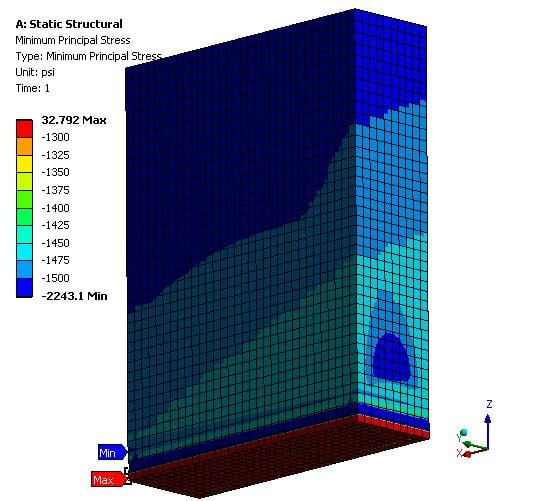The whole bottom layer of elements is red and the stress even goes positive in the corner! The mitigation to this issue is to make another slice that is not included in the stress plot. That is what is shown in the plot below. This result is unpolluted by the fixed support.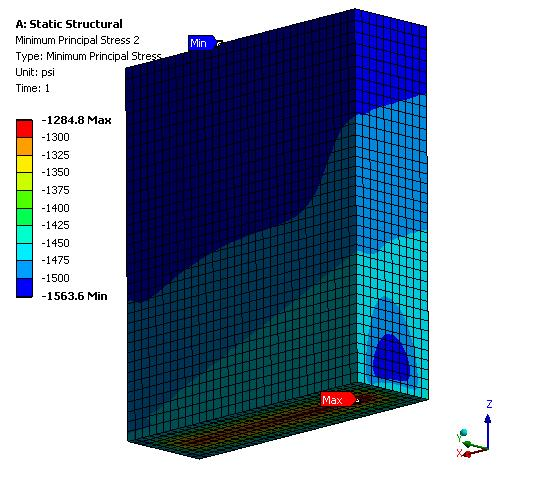I said above that if the pressure magnitudes were equal, then the forces would cancel out. But your equations do not result in equal pressure magnitudes on opposite faces.

I added the Reaction Force for you to examine. When you do, you will see that there is a net sideways force on the slice. Here is the top view of the Reaction Force.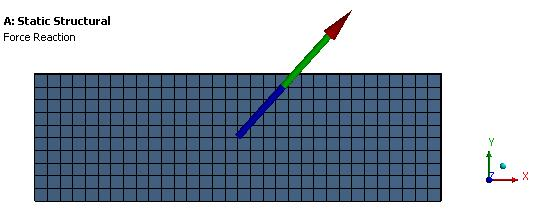The Force Reaction components are Fx = 17E6 N and Fy = 18.8E6 N
The sideways force is thus 25E6 N and the area is 80 m^2 so that is a shear stress of 0.3 MPa. Is that reasonable?

•peteroznewman
Subscriber

Correction: Mohr-Couloub material model is available on the Student license.  I deleted the post where I said it was not. Sorry, that was my mistake, I ran that model on a different computer that did not have the Student license on it.

•Shivani
Subscriber

Hi!

Let me thank you first for all your time and effort that you have put in to help me.

I'm liking working on ANSYS,and you have made it very less stressful.I have a couple of questions.

One: How did you do the second simulation,as shown by you in the  previous post i.e making another slice that is not included in the stress plot.And yes,my answer is fluctuating from negative values to positive values but that should not happen.So your help will be valuable.

Two: Yes,the shear stress value being obtained i.e 0.3 MPa is not reasonable.Does the negative sign on the stress values of the result plot mean that the stress are compressive in nature?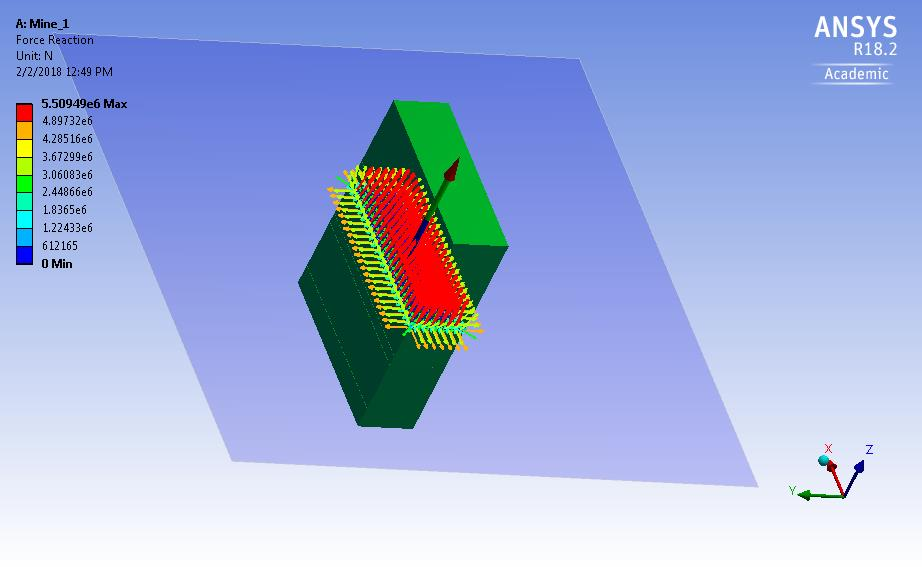Above is my probe for force reaction when I have applied value of pressure in the direction.What does this mean?

•peteroznewman
Subscriber

One: When you create a stress plot, the default is All Bodies. You can type Ctrl-A to select all, then Ctrl-click on the body with the Fixed Support face to remove it from the selection and hit Apply.

Two: Yes, tensile stress is positive, compressive stress is negative.

Looking at the force reaction plot, it appears that you have added a fixed support in the center of your strata, I put it at the bottom. Please attach an archive to your post so I can have a closer look.

•Shivani
Subscriber

In the simulation,I was trying to get similar values of pressures from both direction of x and y (from the formula).But they are slightly different because I can't figure out how exactly should I modify the formula to do that.

•peteroznewman
Subscriber

Shivani, you would benefit from the study of Solid Mechanics where you can learn more about the different stress components. For example, Maximum Principal Stress shows the most positive stress, which is often a tensile stress, while Minimum Principal shows the most negative stress, which in your model is compressive, yet you don't have that in your results.

Equivalent stress is always positive, even when there are only compressive stresses.

I added an extra body to your model so that all your results can plot without being polluted by the fixed support.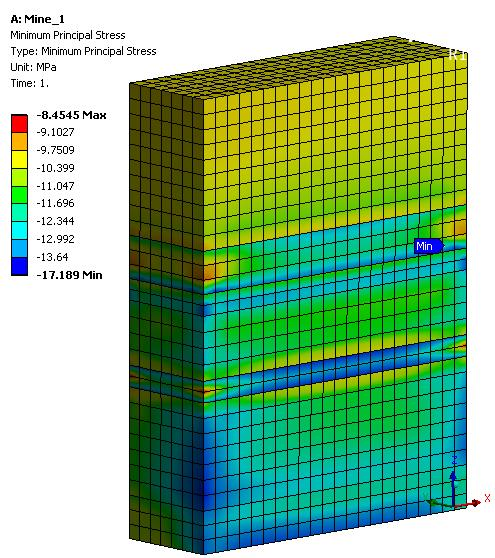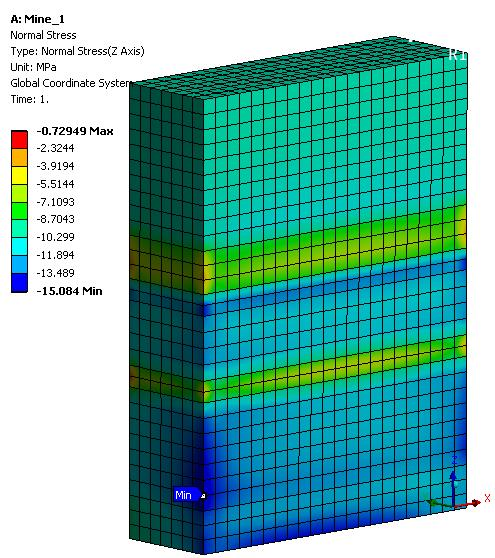I also suppressed the extra pressure equations. You had two X and two Y pressure loads on all the side faces. That is not correct. They just add up.

I have attached my archive.

•Shivani
Subscriber

Hi!

I am familiar with solid mechanics although I feel the need to revisit the course.

Coming to simulation,I think student version does not support changing the number of bodies for stress plot so thank you for doing that.

I had a doubt;since all the three pressures are being distributed across the structure,uniformly then why are the stress contours different on different faces of the model?

•peteroznewman
Subscriber

You can add another body to your model in the Student version, you just have to know the steps to follow. Do you want a video on how to do that?

All three pressures add up on every face (I hope you accounted for that when writing the equations) and the reason different faces show a different contour is because the x or y coordinate is different on each face (for the same z coordinate).  If you make the slice thinner and thinner in the y direction, the pressure contour on the +y face would converge to the same contour that is on the -y face (for the same x, z coordinate). Try it!

•Shivani
Subscriber

Hi again!

Yes,another geometry can be added,but the option to select bodies (of our choice) for stress plot is not getting active.So..I guess that option is not available.

Also I wanted to try simulating it as non linear analysis,would adding plasticity to the engineering data solve the purpose?

And when i add Mohr-Columb properties to the engineering data section,it shows the errors(I have attached the error file for you reference).could you please explain me why are these errors occuring?

My model is suppose to be elastoplastic (a constitutive model).How do I know what kind of model am I simulating?

•peteroznewman
Subscriber

Hi Shivani,

Much Better than a Fixed Support!
I don't know why I didn't think of it before, but the best set of constraints for this problem are these three: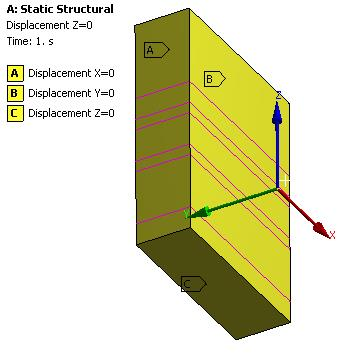That way, there is no artificial stress caused by a Fixed Support.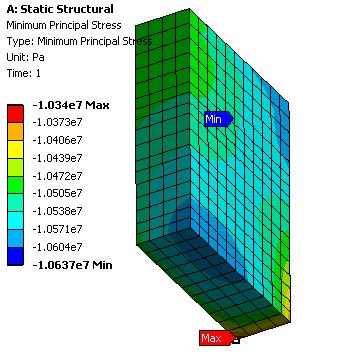To answer your question, when you create a new Stress result, the default is all bodies, but you just pick the body you want. Notice how the extremes on the color legend adjust for just that one body.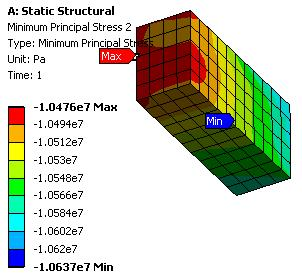Auto Time Stepping
You turn that on when the solver doesn't converge on its own. Otherwise, you can leave it Program Controlled.

Elasto-Plastic Material Model
Plasticity is one of the Material Models in Engineering Data. There are many types. The simplest is Bilinear Kinematic Plasticity. That only requires a Yield Strength and a Tangent Modulus. Perfectly plastic means the Tangent Modulus = 0.
Look at these graphs.

Mohr-Coulomb Material Model
After I deleted the Fixed Support and used the three single axis displacement constraints, the material failure error in the solve.out file did not occur. I suspect that this error occurs when a material model is written assuming that the material will never see tensile stress, because the model becomes undefined in tension, and during the solve, a tensile stress is calculated, the solver has to stop with an error.

•Shivani
Subscriber

Looks like this is done for now.

Because they(where I have to submit the simulation) have changed the conditions.So maybe I'll see in another thread!

You have been a good help indeed.Thank you.

•Shivani
Subscriber

Hi!

I have the simulation of the mining model (this time with a gallery).I have attached simulation of two models.In one I have given pressure in three directions and in one I have suppressed two pressure and still my result is the same.I wanted to know the reason for this.

All the pressure are variable in the 'z' direction (function of height).

•peteroznewman
Subscriber

Hi Shivani,

There was an error after I restored the attached file, but I was able to recover by Export/Import of the first system.

Mistakes in Model.
1. There is a Displacement of X=0 on OPPOSITE sides of the block. It should only be on one side. The X=0 displacement means that the pressure on that face can have no effect.

2. There is a Displacement of Y=0 on OPPOSITE sides of the block. It should only be on one side. The Y=0 displacement means that the pressure on that face can have no effect.

3. Pressure BC is set on all faces, so there is no need for Pressure Y and Pressure X since they use the same formula.

4. There is a Fixed Support but you have three Displacement BCs that make a Fixed Support unnecessary and harmful to the result.

5. The Displacement BC I renamed Displacement Z and set Z=0 and X and Y free.

6. Pressure BC is set on the sides of the tunnel, but that is air, not rock. There is no pressure on the tunnel walls.

Questions.
What units are the equations for pressure written in?  If they are MPa, then the units should be mm, but if they are Pa, then the units should be m. I changed the units to m.  Be very careful to have the units set correctly before a Solve.

Other Changes to the Model
I turned on Auto Time Stepping and set the Initial Substeps to 10.  I set the solver to Direct.

Now you do get a different result when Pressure Y and X are unsuppressed, but it is not correct to apply the same pressure to a face twice. Either delete Pressure Y and X or change Pressure to apply to only the top face.

•Romaric
Subscriber
n